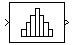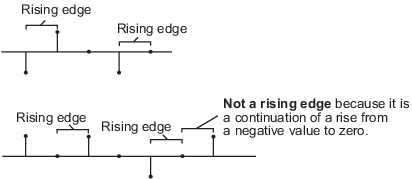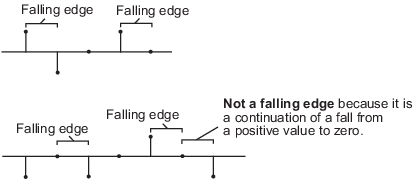# 2-D Histogram

Generate histogram from input

•Libraries:
Computer Vision Toolbox / Statistics

## Description

The 2-D Histogram block computes the frequencies of the elements in the input image. The block calculates the histogram values for either the entire input or for each column of the input image. It sorts all the of input values into bins according to their pixel values. The histogram value for a given bin represents the frequency of the input values bracketed by that bin. The block is also capable of computing a running histogram across a sequence of inputs. The block is also capable of computing a running histogram across a sequence of inputs.

## Ports

### Input

expand all

Input image, specified as a vector, matrix, or 3-D array.

Data Types: `single` | `double` | `int8` | `int16` | `int32` | `uint8` | `uint16` | `uint32` | `fixed point`

Reset value for an input sequence, specified as a scalar. This value dictates the event that causes the block to reset the running histogram. The sample time of the Rst input must be a positive integer and a multiple of the input sample time.

#### Dependencies

To enable this port, select the Running histogram parameter and set the Trigger type parameter to ```Rising edge```, `Falling edge`, ```Either edge```, or `Non-zero sample`.

Data Types: `single` | `double` | `int8` | `int16` | `int32` | `uint8` | `uint16` | `uint32` | `Boolean` | `fixed point`

### Output

expand all

Histogram output, returned as a vector, matrix, or 3-D array.

If the Find the histogram over parameter is set to `Entire input`, then the output is always a vector. If the Find the histogram over parameter is set to ```Each column```, then the output has the same number of dimension as the input.

Data Types: `single` | `double` | `uint32`

## Parameters

expand all

Main

Specify a real-valued scalar for the lower boundary, Bm, of the lowest-valued bin. `NaN` and `inf` are not valid values for Bm.

Tunable: Yes

Specify a real-valued scalar for the upper boundary, Bm, of the highest-valued bin. `NaN` and `inf` are not valid values for BM.

Tunable: Yes

Specify the number of bins in the histogram.

Specify the dimension of the input image along which the block computes the histogram values. For any given value, n, of the Number of bins parameter, this parameter affects the output of the block as follows.

• `Entire input` — The block computes the histogram over the entire input. It outputs an n- element column vector.

• `Each column` — The block computes the histogram over each column of the input.

• If the input is an M-by-N matrix, the block outputs an n-by-N matrix. The jth column of the output matrix contains the histogram for the data in the jth column of the M-by-N input matrix.

• If the input is an M-by-N-by-P array, the block outputs an n-by-N-by-P array. If the input is a three-channel color image, the value of P is 3, and the block computes the histogram for each color channel separately.

Select the Normalized parameter to normalize the output histogram value within a range of [0, 1].

Note

Use of this parameter is not supported for fixed-point signals.

Select the Running histogram parameter to compute the frequency distribution of both the past and present data for successive inputs. The block resets the histogram (by emptying all of the bins) when it detects a reset event at the optional Rst port. There's an additional dimension to the output size which is equal to ((Tsim/Ts)+1) where Tsim is the simulation time and Ts is the sample time for block execution. The output depends on the value of the Find the histogram over parameter.

• `Entire input` — The block computes a running histogram for the data in the first dimension of the input. The block outputs an n-by-1-by-((Tsim/Ts)+1), where n is the value of Number of bins parameter.

• `Each column` — The block computes a running histogram for each column of the M-by-N matrix. The block outputs an n-by-N-by-((Tsim/Ts)+1) array, where n is the value of the Number of bins parameter.

Note

When the block is used in running mode and the input data type is non-floating point, the output of the histogram is stored as a `uint32` data type. The largest number that can be represented by this data type is `232–1`. If the range of the `uint32` data type is exceeded, the output data wraps back to 0.

Specify the event that, when detected at the optional Rst port, resets the running histogram. The reset signal and the input data signal must have the same rate.

Specify the reset event as:

• `None` — Disables the Rst port

• `Rising edge` — Triggers a reset event when the Rst input does one of the following:

• Rises from a negative value to either a positive value or zero

• Rises from zero to a positive value, where the rise is not a continuation of a rise from a negative value to zero• `Falling edge` — Triggers a reset event when the Rst input does one of the following:

• Falls from a positive value to either a negative value or zero

• Falls from zero to a negative value, where the fall is not a continuation of a fall from a positive value to zero• `Either edge` — Triggers a reset event when the Rst input is a rising edge or falling edge

• `Non-zero sample` — Triggers a reset event at each sample time that the Rst input is not zero

#### Dependencies

To enable this parameter, select the Running histogram parameter.

Data Types

For details on the fixed-point block parameters, see Specify Fixed-Point Attributes for Blocks.

Select this parameter to prevent the fixed-point tools from overriding the data types you specify in this block. For more information, see Lock the Output Data Type Setting (Fixed-Point Designer).

## Block Characteristics

 Data Types `double` | `fixed point` | `integer` | `single` Multidimensional Signals `no` Variable-Size Signals `no`

## Algorithms

The histogram bins have equal width of:

`$\Delta =\frac{{B}_{M}-{B}_{m}}{n},$`

where

• BM — Represents the upper boundary of the highest-valued bin.

• Bm — Represents the lower boundary of the lowest-valued bin.

• n — Represents the number of bins.

The centers are located at:

`${B}_{m}+\left(k+\frac{1}{2}\right)\Delta \begin{array}{cccc}& & & k=0,1,2,...,n-1\end{array}.$`

For input values that fall between two bins, the block places them in the lower-valued bin. For input values greater than the upper limit of the histogram or less than the lower limit of the histogram, the block places them in the highest-valued or lowest-valued bin, respectively.

## Version History

Introduced before R2006a